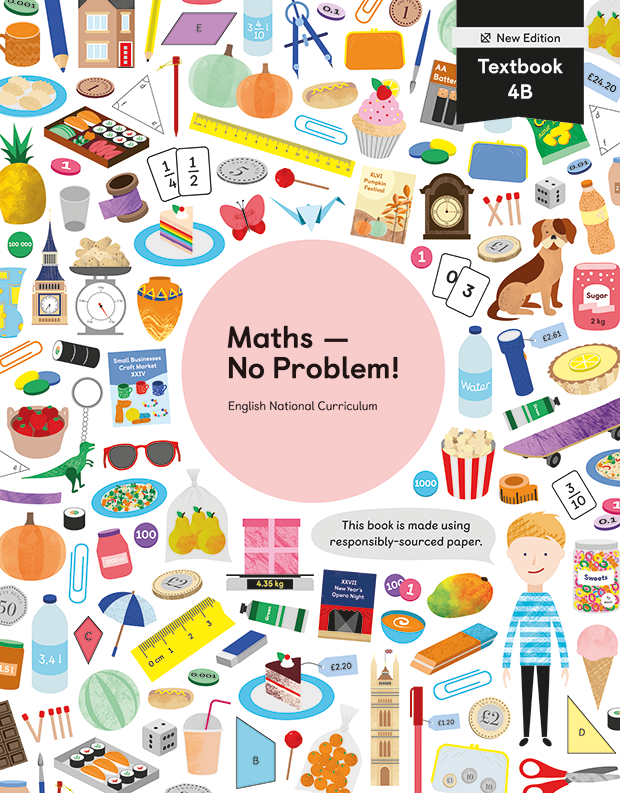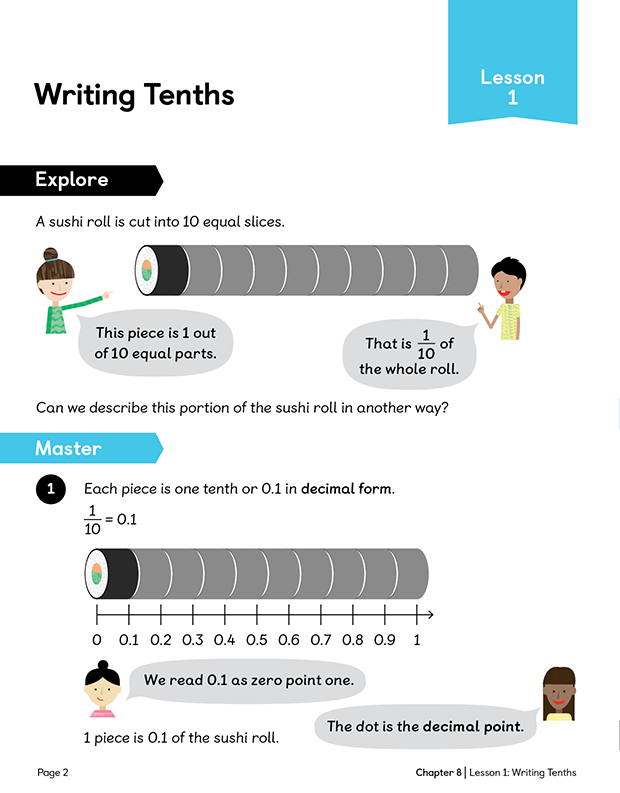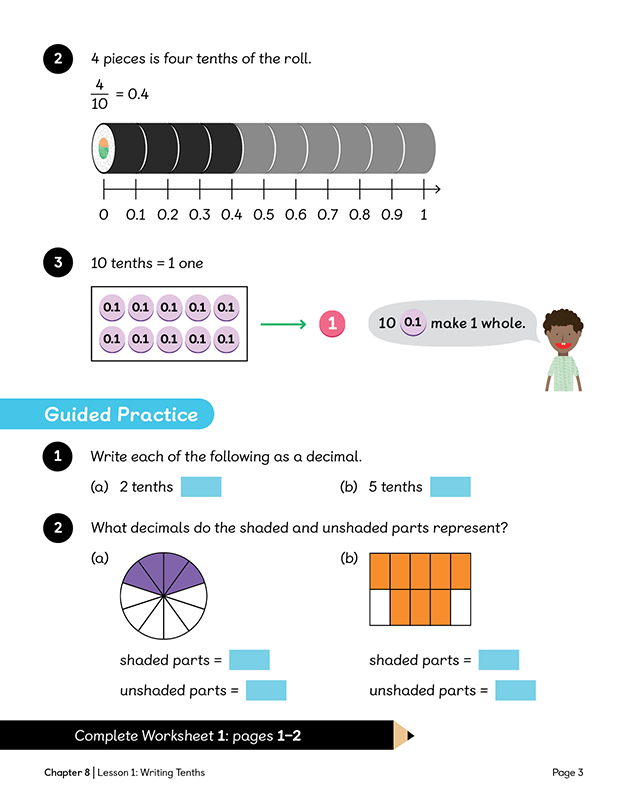Textbook 4B New Edition# Maths — No Problem!Textbook 4B New Edition

## £11.49

ISBN: 9781913458607
Pages: 192
Availability:
In stock
Qty:

• Lesson 1 - Writing TenthsTo be able to recognise and write decimal equivalents of any number of tenths.
• Lesson 2 - Writing TenthsTo be able to recognise and write decimal equivalents of any number of tenths.
• Lesson 3 - Writing HundredthsTo be able to recognise and write decimal equivalents of any number of hundredths.
• Lesson 4 - Writing HundredthsTo be able to recognise and write decimal equivalents of any number of hundredths.
• Lesson 5 - Dividing by 10 and 100To be able to divide 1-digit and 2-digit numbers by 100.
• Lesson 6 - Making Number PatternsTo be able to add or subtract tenths and hundredths from a decimal.
• Lesson 7 - Comparing and Ordering DecimalsTo be able to compare and order numbers with the same number of decimal places up to 2 decimal places.
• Lesson 8 - Rounding DecimalsTo be able to round numbers with 1 decimal place to the nearest whole number.
• Lesson 9 - Writing Fractions as DecimalsTo be able to recognise and write decimal equivalents 1/4, 1/2 and 3/4.
• Chapter ConsolidationTo be able to apply knowledge of decimal numbers to solve problems.

## Author(s)

Consultant Editors: Dr Wong Khoon Yoong, Dr Julie Alderton
Series Editors: Adam Gifford, Andy Psarianos